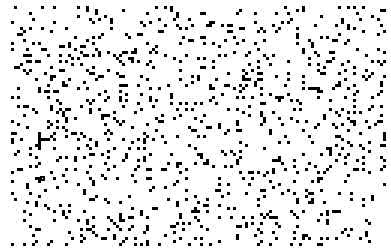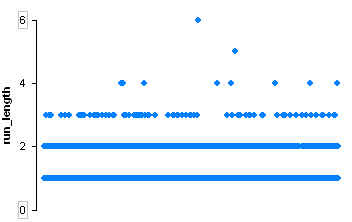## Thursday, March 26, 2009

### Pi's first six in a row

If you look at the 'pi color map' and see a vertical stripe, what you are seeing is a run of repeated digits, like 7777, 00000, or 222.

One occurs in the top left:Sequences like this used to be important examples in motivating discussions about the law of the excluded middle. Statements like: 'the decimal expansion of pi contains the sequence 7777', according to the law of the excluded middle, are either true or false. But when you are discussing something that has never been calculated, and that might never be calculated, suggests (to constructivists), that the statement may be neither true or false, and that the sequence 7777 may be somewhat like Schrodinger's cat, neither existing nor not existing.

Now that the digits of pi have been calculated to astounding lengths, and that mathematics exists to uncover the properties the pi digit sequence, the expansion of pi is no longer an ideal candidate for constructivist thought experiments. Even some exotic questions about the decimal expansion of pi, like the convergence of the brower-heyting sequence, have been resolved. Questions about the expansion of pi that illustrate constructivist problems can still be formulated, but they need to be more complicated than they used to be.

Still, I decided to look more closely at this little sequence that occurs (relatively) early on in the pi expansion. The stripe in the pi colour map mentioned above corresponds to the sequence 999999 beginning at digit 763 and ending at digit 768. This was observed in a set of the first 2281 digits of pi.

I decided to push the software a bit further, and load 10000 digits of pi into the document.  (A file with the first 10000 digits of pi formatted for Fathom or TinkerPlots is here.) The plot below shows the digits with 9 in black and all other digits blanked out - dots are single occurrences of a 9, while vertical stripes are runs of repeated 9s.Using Tinkerplots' runLength function we can see how frequent runs of various lengths are, and easily identify the longer ones.Even with 10000 digits, the 999999 sequence at digit 763 was still the longest sequence of repeated digits. The other runner-up sequences in this first 10000 digits are, two instances of 2222, a single run of 8888, and four distinct occurrences of 7777.

Interestingly, an obituary for pre-digital-computer pi-expander John Wrench was posted today on the MAA Math DL site

The plot at the bottom of this post shows the distribution of even and odd digits in the decimal expansion of pi's first 10000 digits, and was inspired by a similar plot from Wolfram Math World.